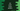# Different ways in C# to print a cross pattern## C# program to print a cross pattern:

This post will show you how to print a cross star pattern in C# using any character. We will learn the algorithm and how to print the pattern programmatically in different ways.

The program will take the size of the pattern as an input from the user and print it to the user.

It looks as like below:

``````*     *
*   *
* *
*
* *
*   *
*     *``````

Let’s learn the algorithm first.

### Algorithm to print a cross star pattern:

Let’s learn how to print a cross star pattern. For example, suppose we want to print the below pattern:

``````*     *
*   *
* *
*
* *
*   *
*     *``````

* and blank spaces are used to print this pattern. If you replace all blank spaces with #, it will look as like below:

``````*#####*
#*###*#
##*#*##
###*###
##*#*##
#*###*#
*#####*``````
• Total rows are 7 and total columns are 7.
• For row 1, * is printed at column 1 and 7.
• For row 2, * is printed at column 2 and 6.
• For row 3, * is printed at column 3 and 5.
• For row 4, * is printed at column 4
• For row 5, * is printed at column 5 and 3 etc.

So, * is printed if the values of row and column are equal. Also if i + j is equal to height + 1. For other positions, we have to print a blank space.

### Example 1: C# program to print a cross pattern with star:

Let’s write down the program:

``````namespace Program
{
class Program
{
static void Main(string[] args)
{
int Size;

Console.WriteLine("Enter the size: ");

Console.WriteLine();

for (int Row = 1; Row <= Size; Row++)
{
for (int Column = 1; Column <= Size; Column++)
{
if (Row == Column || Row + Column == Size + 1)
{
Console.Write("*");
}
else
{
Console.Write(" ");
}
}
Console.WriteLine();
}

}
}
}``````

Here,

• The size of the pattern is stored in the variable Size.
• Two for loops are used to print the pattern. Both of these loops runs for Size number of times. The outer loop runs from Row = 1 to Row = Size and the inner loop runs from Column = 1 to Column = Size.
• Inside these loops, we are printing * if Row is equal to Column or Row + Column is equal to Size + 1. Else, it prints a blank space.
• At the end of each iteration of the outer loop, i.e. after each row is printed, it adds a new line.

If you run this program, it will give output as like below:

``````Enter the size:
9

*       *
*     *
*   *
* *
*
* *
*   *
*     *
*       *``````

If the height is even, it will look as like below:

``````Enter the size:
10

*        *
*      *
*    *
*  *
**
**
*  *
*    *
*      *
*        *``````

### Example 2: C# program to print a cross pattern with star and while loop:

We can also use while loops to print the same cross pattern instead of for loops. There won’t be any change to the algorithm.

``````namespace Program
{
class Program
{
static void Main(string[] args)
{
int Size, Row = 1, Column;

Console.WriteLine("Enter the size: ");

Console.WriteLine();

while (Row <= Size)
{
Column = 1;
while (Column <= Size)
{
if (Row == Column || Row + Column == Size + 1)
{
Console.Write("*");
}
else
{
Console.Write(" ");
}
Column++;
}
Console.WriteLine();
Row++;
}

}
}
}``````

The integer variable Row is initialized as 1 and Column is another integer variable.

At the end of each iteration of the while loop, the value of Row is incremented by 1. Also the Column value is incremented at the end of each iteration of the inner while loop.

A new line is printed at the end of each iteration of the outer while loop. It will print similar result.

``````Enter the size:
11

*         *
*       *
*     *
*   *
* *
*
* *
*   *
*     *
*       *
*         *``````